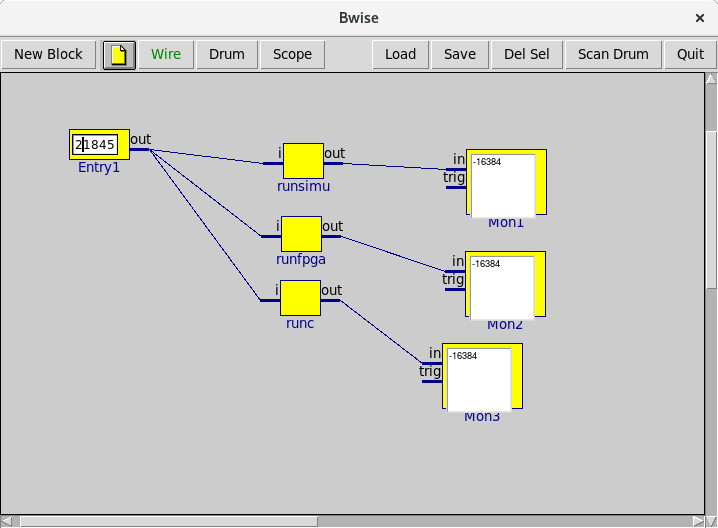## Bwise blocks connecting FPGA accelerated C functions

TV As a worthy addition to the BWise examples of using graphical flow based programming with Tcl/Tk I demonstrate a C function connected with a BWise flow being transformed into a FPGA program, connected with a live BWise graph and run on an embedded Linux system. The A.R.M. based Parallella board (see Bwise on the Parallella) can also run the bwise application and can run for instance mat problems competitive with desktop computer speeds.

I use the latest Xilinx tools for Silicon Compilation, which happen to completely run from Tcl commands. The C function being transformed into an FPGA image dynamically loaded into the Zynq 7010 board is automatically turned into an AXIlite bus connected Verilog function by Vivado_hls, which requires little adaptation to a regular, complex C function with variables transferred by name, allowing any C control structure and includes C library functions like math calls.

Here's the first experiment with the actual FPGA board connecting with Tcl: A Tcl extension for FPGA compiled C function direct interaction. That page explains a bit of the software technology, and arrives at three main tests for seeing if the C to FPGA compiled function has made it properly into a tcl extension driven (test) application. A formula to maxima evaluation of the input number, a C compiled to a regular CPU function version, and the Tcl extension driven FPGA function. See Hello World as a C extension for a simpler tcl extension example.

Those three methods of checking out our sine table initialization and testing, Maxima well formed formula, C function (with the exact same C function that was compiled to FPGA by the free Xilinx tool set) and actual FPGA implementation can be compared using BWise blocks, driven by the same test value, input as an integer in text form. The Maxima (see e.g. Bwise blocks using exec maxima) interpreter takes an integer in text form, interprets it as integer, computes the desired lookup value, and returns it in text integer form. The C program simply reads an argument from the command line and converts it from text to a short integer, like used by the example function. The FPGA lookup and initialization (once when the programmable logic image is loaded in is addressed by the Zynq boards' A.R.M. CPUs over AXI-lite bus the first time) sets up the IO to and from the FPGA logic to be under control of the Tcl extension (see above link), so it's possible to pass a integer that got converted from text to a machine integer over the device register communication without extra copies or conversions, and the same for the return value.

So to get a test BWise canvas going, we need the tcl functions from the Tcl FPGA extension page above, and a new one for the C function simulation by an actual normal C program:

``` proc runc { {i} } {
return [exec ./testsinf \$i]
} ```

And we make these 3 different "run" procedures into bwise blocks with one input and one output (a string representing a short integer):

``` proc_toblock runfpga
proc_toblock runc
proc_toblock runsimu```

We add an input entry and some monitors:So now, we can type in values in the entry on the left, and see what the different function implementations from Maxima math, C, and FPGA-C yield as output in practice, running bwise on the devboard with the fpga and maxima installed. We can also set the input integer smart, like at (-)Pi/6 :

``` set Entry1.out [expr int(32768 - (32768 / 3.0))]
net_funprop Entry1```

Using maxima we know:

``` domaxima "solve(sin(x)=1/2,x)"
: using arc-trig functions to get a solution.
Some solutions will be lost.
(%o2) [x = %pi/6]```

So that the outcome should be 1/2 from the full range of short integer values, and all blocks agree to this.

 Category Bwise